# Foundations of Robotics (WIP)

If you find any error you can edit article using edit link $\uparrow$.

• Karel Čapek: R.U.R.
• Isaac Asimov: Runaround
• B. Siciliano, O. Khatib: Handbook of Robotics
• S.M. LaValle: Planning Algorithms
• R.J. Schilling: Fundamentals of robotics: Analysis & Control

## History

Robots are old and in movies. There is also robotic fish.

### Prehistory

There is book Leonardos robots. Japan had mechanic dolls.

### Robotic structures

• Manipulators
• 40’s of 20$^{\text{th}}$ century - remote manipulation with radioactive material
• 1961 - H.A. Ernst (MIT) - founder of company making manipulator robots
• Mobile robots - remotely controlled
• since ca. 70’s of 20$^{\text{th}}$ century
• Mobile robots - autonomous
• since ca. 90’s of 20$^{\text{th}}$ century

### Three laws of robotics

1. Law
A robot may not injure a human being or, through inaction, allow a human being to come to harm

2. Law
A robot must obey orders given to it by human beings, except where such orders would conflict with the first law.

3. Law
A root must protect its own existence as long as such protection does not conflict with the first or second laws.

by Isaac Asimov, 1942, Runaround

## Kinematics

• Motion and transformation
• translation, rotation, spherical movement
• Movement of individual robot parts without relation to forces which move it.
• Representation of the location and orientation in space
• Forward vs. Inverse kinematics
• Calculate position of end effector vs. Calculate motion to reach desired position

### Degrees of freedom (DOF)

• Basic directions of translation and rotation
• 2D - 3 DOF [$x,y,\alpha$]
• 3D - 6 DOF [$x,y,z,\alpha,\beta,\gamma$]
• Alternatively “$x,y,z$ position + rotation i.r.t $xy$ and $zy$ planes and i.r.t. the tool rotation axis”
• Human body has 244 DOF

### Manipulators

• Manipulation of an object in space
• at least 6 DOF for 3D
• Arms, elbows, joints, hinges
• Joint variable $q_i$
• denotes joint setup, orientation
• Joints State $q = [q_1, q_2,...,q_n]$
• DOF = $n$
• Working space
• Local vs. Global coordinate system (LCS, GCS)
• coordinates transformation
• lokální je užitečnější

### Lower pair joints

• Many variants possible, but usually:
1. revolute (DOF = 1)
2. prismatic (DOF = 1)
3. helical (DOF = 1)
4. cylindrical (DOF = 2)
5. spherical (DOF = 3)
6. planar (DOF = 3)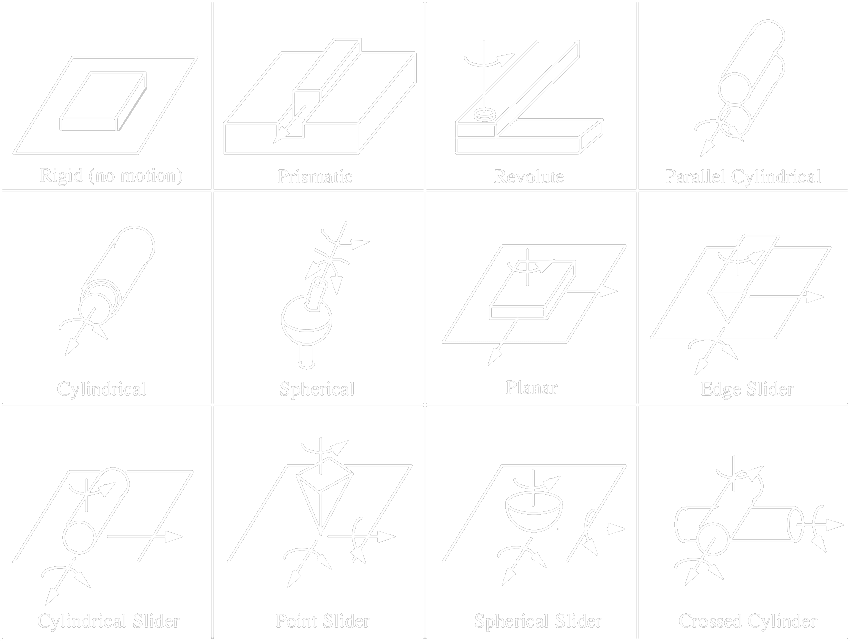• Primitive joint types:
• prismatic
• revolute
• Most robots are build using only these two

### Rotation

$P' = R . P$

#### Rotation $x$ axis, angle $\phi$

$R_{x, \phi} = \begin{bmatrix} 1 & 0 & 0 \\ 0 & \cos\phi & -\sin\phi \\ 0 & \sin\phi & \sin\phi \end{bmatrix}$

#### Rotation $y$ axis, angle $\psi$

$R_{y, \psi} = \begin{bmatrix} \cos\psi & 0 & \sin\psi \\ 0 & 1 & 0 \\ -\sin\psi & 0 & \sin\psi \end{bmatrix}$

#### Rotation $z$ axis, angle $\xi$

$R_{z, \xi} = \begin{bmatrix} \cos\xi & -\sin\xi & 0 \\ \sin\xi & \sin\xi & 0 \\ 0 & 0 & 1 \\ \end{bmatrix}$

#### Rotation along $x$,$y$,$z$ by angles $\phi$, $\psi$, $\xi$

$R_{\phi, \psi, \xi}=\left[\begin{array}{ccc} \cos (\psi) \cos (\xi) & -\cos (\psi) \sin (\xi) & \sin (\psi) \\ {} & {} & {} \\ \sin (\phi) \sin (\psi) \cos (\xi) & -\sin (\phi) \sin (\psi) \sin (\xi) & \\ +\cos (\phi) \sin (\xi) & +\cos (\phi) \cos (\xi) & -\sin (\phi) \cos (\psi) \\ & & \\ -\cos (\phi) \sin (\psi) \cos (\xi) & \cos (\phi) \sin (\psi) \sin (\xi) & \cos (\phi) \cos (\psi) \\ +\sin (\phi) \sin (\xi) & +\sin (\phi) \cos (\xi) & \end{array}\right]$

Bod $p = (x,y,z,w)^{T}, x,y,z \in \R, w = 1$

### 2D Rotation

$\left(x^{\prime}, y^{\prime}, w^{\prime}\right)^{T}= \left[\begin{array}{ccc} \cos (\alpha) & -\sin (\alpha) & 0 \\ \sin (\alpha) & \cos (\alpha) & 0 \\ 0 & 0 & 1 \end{array}\right] \begin{bmatrix}x \\ y \\ w\end{bmatrix}$

### 2D Translation

$\left(x^{\prime}, y^{\prime}, w^{\prime}\right)^{T}= \left[\begin{array}{ccc} 1 & 0 & d_{x} \\ 0 & 1 & d_{y} \\ 0 & 0 & 1 \end{array}\right] \begin{bmatrix}x \\ y \\ w\end{bmatrix}$

### Rotation + translation

$P^{\prime}=R \cdot P + T \rightarrow P^{\prime} = \Tau . P$

$\begin{bmatrix} P' \\ 1 \end{bmatrix} = \begin{bmatrix} R & T \\ 0\cdots0 & 1 \end{bmatrix} \cdot P$ $\begin{bmatrix} x' \\ y' \\ z' \\ 1 \end{bmatrix} = \begin{bmatrix} \begin{bmatrix} \square & \square & \square \\ \square & \square & \square \\ \square & \square & \square \\ \end{bmatrix} &\kern-1em \begin{array}{c} t_{x} \\ t_{y} \\ t_{z} \end{array} \\ 0 \kern1.3em 0 \kern1.3em 0 &\kern-1em 1 \end{bmatrix} \cdot \begin{bmatrix} x \\ y \\ z \\ 1 \end{bmatrix}$

### Forward kinematics (3D)

• $P = f(q)$
• $q = [q_{1},q_{2},\ldots,q_{6}]$
• $P = [x,y,z,\alpha,\beta,\gamma]$
• General
• each link represented by its geometric transformation
• transition between $LCS$s (local coordinates system)
• may be hard to construct the full combined transformation matrix

### System composition

• General a. each link represented by its geometric transformation b. translation between $LCS$s
• May be hard to construct the full combined transformation matrix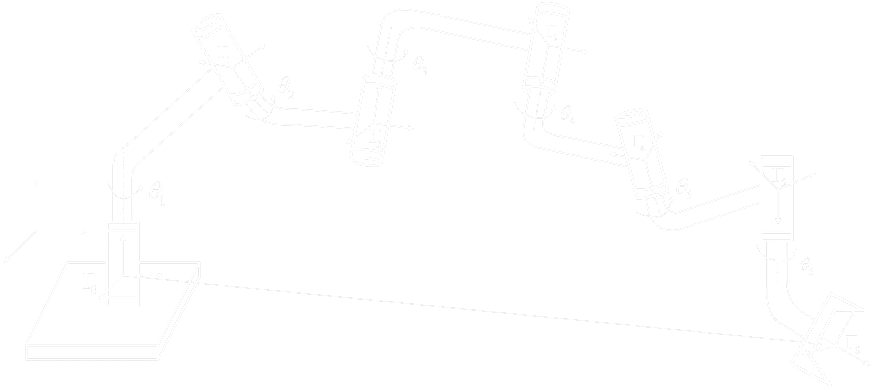### Denavit-Hartengerg

Fictive movements which connect two systems:

rotate, move, move, rotate.

That can be generalized to any sequence.

• Typical system composition
• Chains composed of rotational and translatioal joints only:
• joint $k$ connects links $k_{i-1}$ and $k_i$
• link $k$ connects joints $k_i$ and $k_{i+1}$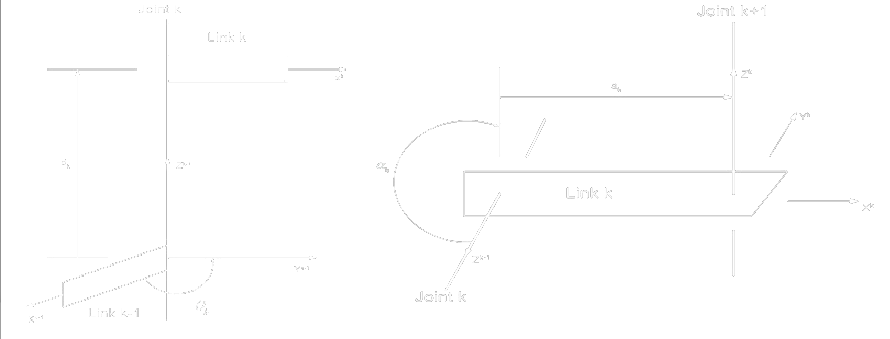##### Denavit-Hartenberg overview

Definice

Relation between $L C S_{i-1}$ and $L C S_{i}$ is a composed transformation:

 1. Rotate $x_{i-1}$ axis along $z_{i-1}$ by angle $\vartheta_{i}$ $A_{z_{i-1},\vartheta_{i}}$ 2. Translate $x_{i-1}$ axis towards $z_{i-1}$ by distance $d_{i}$ $A_{z_{i-1}, d_{i}}$ 3. Translate $L C S_{i-1}$ along $x_{i}$ axis by distance $a_{i}$ $A_{x, a_{i}}$ 4. Rotate $z_{i-1}$ axis along $x_{i}$ by angle $\alpha_{i}$ $A_{x, \alpha_{i}}$

DH parameters: $\vartheta_{i}, d_{i}, a_{i}, \alpha_{i}$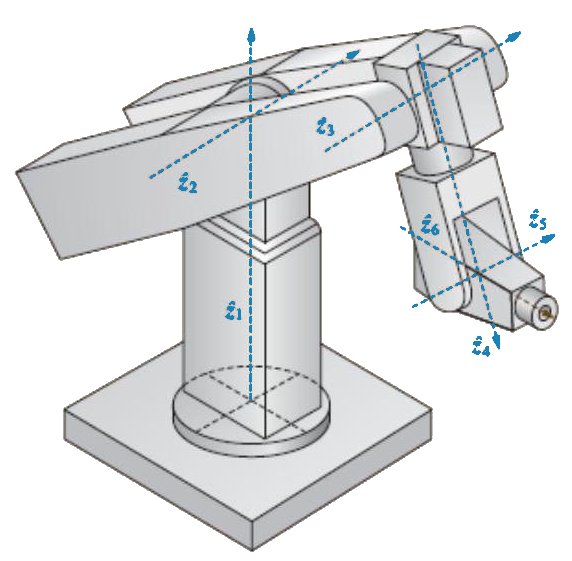#### DH transformation

1. Rotate $x_{i-1}$ axis along $z_{i-1}$ by angle $\vartheta_{i}$

$A_{z_{i-1}, \vartheta_{i}}=\left[\begin{array}{cccc} \cos \left(\vartheta_{i}\right) & -\sin \left(\vartheta_{i}\right) & 0 & 0 \\ \sin \left(\vartheta_{i}\right) & \cos \left(\vartheta_{i}\right) & 0 & 0 \\ 0 & 0 & 1 & 0 \\ 0 & 0 & 0 & 1 \end{array}\right]$
2. Translate $x_{i-1}$ axis towards $z_{i-1}$ by distance $d_{i}$

$A_{z_{i-1}, d_{i}}=\left[\begin{array}{cccc} 1 & 0 & 0 & 0 \\ 0 & 1 & 0 & 0 \\ 0 & 0 & 1 & d_{i} \\ 0 & 0 & 0 & 1 \end{array}\right]$
3. Translate $L C S_{i-1}$ along $x_{i}$ axis by distance $a_{i}$

$A_{x_{i}, a_{i}}=\left[\begin{array}{cccc} 1 & 0 & 0 & a_{i} \\ 0 & 1 & 0 & 0 \\ 0 & 0 & 1 & 0 \\ 0 & 0 & 0 & 1 \end{array}\right]$
4. Rotate $z_{i-1}$ axis along $x_{i}$ by angle $\alpha_{i}$

$A_{z_{i-1}, d_{i}}=\left[\begin{array}{cccc} 1 & 0 & 0 & 0 \\ 0 & \cos \left(\alpha_{i}\right) & -\sin \left(\alpha_{i}\right) & 0 \\ 0 & \sin \left(\alpha_{i}\right) & \cos \left(\alpha_{i}\right) & 0 \\ 0 & 0 & 0 & 1 \end{array}\right]$

Finálně vše zkombinujeme

$A_{i-1}^{i}=A_{z_{i-1}, \vartheta_{i}} \cdot A_{z_{i-1}, d_{i}} \cdot A_{x, a_{i}} \cdot A_{x, \alpha_{i}}$ $A_{i-1}^{i}= \left[\begin{array}{cccc} \cos \left(\vartheta_{i}\right) & -\sin \left(\vartheta_{i}\right) \cos \left(\alpha_{i}\right) & \sin \left(\vartheta_{i}\right) \sin \left(\alpha_{i}\right) & a_{i} \cos \left(\vartheta_{i}\right) \\ \sin \left(\vartheta_{i}\right) & \cos \left(\vartheta_{i}\right) \cos \left(\alpha_{i}\right) & -\cos \left(\vartheta_{i}\right) \sin \left(\alpha_{i}\right) & a_{i} \sin \left(\vartheta_{i}\right) \\ 0 & \sin \left(\alpha_{i}\right) & \cos \left(\alpha_{i}\right) & d_{i} \\ 0 & 0 & 0 & 1 \end{array}\right]$
• DH parameters: $\vartheta_{i}, d_{i}, a_{i}, \alpha_{i}$
• $\vartheta_{i}$ angle between $x$ axes about $z_{i-1}$ (joint angle)
• $d_{i}$ distance between $x$ axes (link offset)
• $a_{i}$ distance between $z$ axes (link length)
• $\alpha_{i}$ angle between $z$ axes about $x_{i}$ (link twist)

#### $A^i_{i-1}$ usage

• universal transformation between two adjacent $LCS$
• Same format independent to joint type:
• Rotation - $\vartheta_{i}$ variable, others are constant
• Traslation - $d_{i}$ variable, others are constant
• Forward kinematics is easy:
• while iterating, we use always one variable and 3 constants

#### DH system construction

• Joints numbered $0\ldots n$ ($0$ is the first, fixed, $1\ldots n$ are the rest, moving)
• Links numbered $1\ldots n$ (link $i$ connects joints $i-1$ and $i$)
• Right-handed orthonormal coordinate system
• Let axis $z_{i-1}$ be the axis of joint $i$ movement, positive direction towards positive quadrant of the basic system
• Let axis $x_{i}$ be perpendicular to $z_{i-1}$ and $z_{i}$ :
• $z_{i-1}$ and $z_{i}$ identical - endpoint of joint $i$, parallel to $x_{i-1}$
• skew $-x_{i}$ share the normal $z_{i-1}$ a $z_{i}$, positive direction from $z_{i-1}$ towards $z_{i}$.
• intersecting $-x_{i}$ perpendicular to $z_{i-1}$ and $z_{i}$, in the intersection, positive direction so that it moves along $x_{i}$ from $z_{i-1}$ to $z_{i}$ in positive sense
• Set $y_{i}$ axis to complete the right-handed orthonormal $LCS_{i}$
• Set $LCS_{i}$ origin at intersection of $z_{i-1}$ and $z_{i}$ or (if they do not intersect) at intersection of their common normal and $z_{i}$
• Determine the four parameters:
• $\vartheta_{i} \ldots$ angle of rotation from $x_{i-1}$ to $x_{i}$ about $z_{i-1}$
• $d_{i} \ldots$ distance from $L C S_{i}$ origin to $b_{i}$ along $z_{i-1}, b_{i}$ is the intersection of $x_{i}$ and $z_{i-1}$ (or $x_{i}$ and their common normal)
• $a_{i} \ldots$ distance from $b_{i}$ to $L C S_{i}$ origin along $x_{i}$
• $\alpha_{i} \ldots$ angle of rotation from $z_{i-1}$ to $z_{i}$ about $x_{i}$
• $z_{n}$ from the endpoint of last link either parallel to $z_{n-1}$ or to some significant direction (e.g. supply cable)
• $x_{n}$ from the endpoint of the last link so that it intersects $z_{n-1}$, positive direction towards the workspace.

## Motion model

### Holonomic / non-holonomic drive

Definice

A vehicle is holonomic if the number of local degrees of freedom of movement equals the number of global degrees of freedom.

### Ackermann steering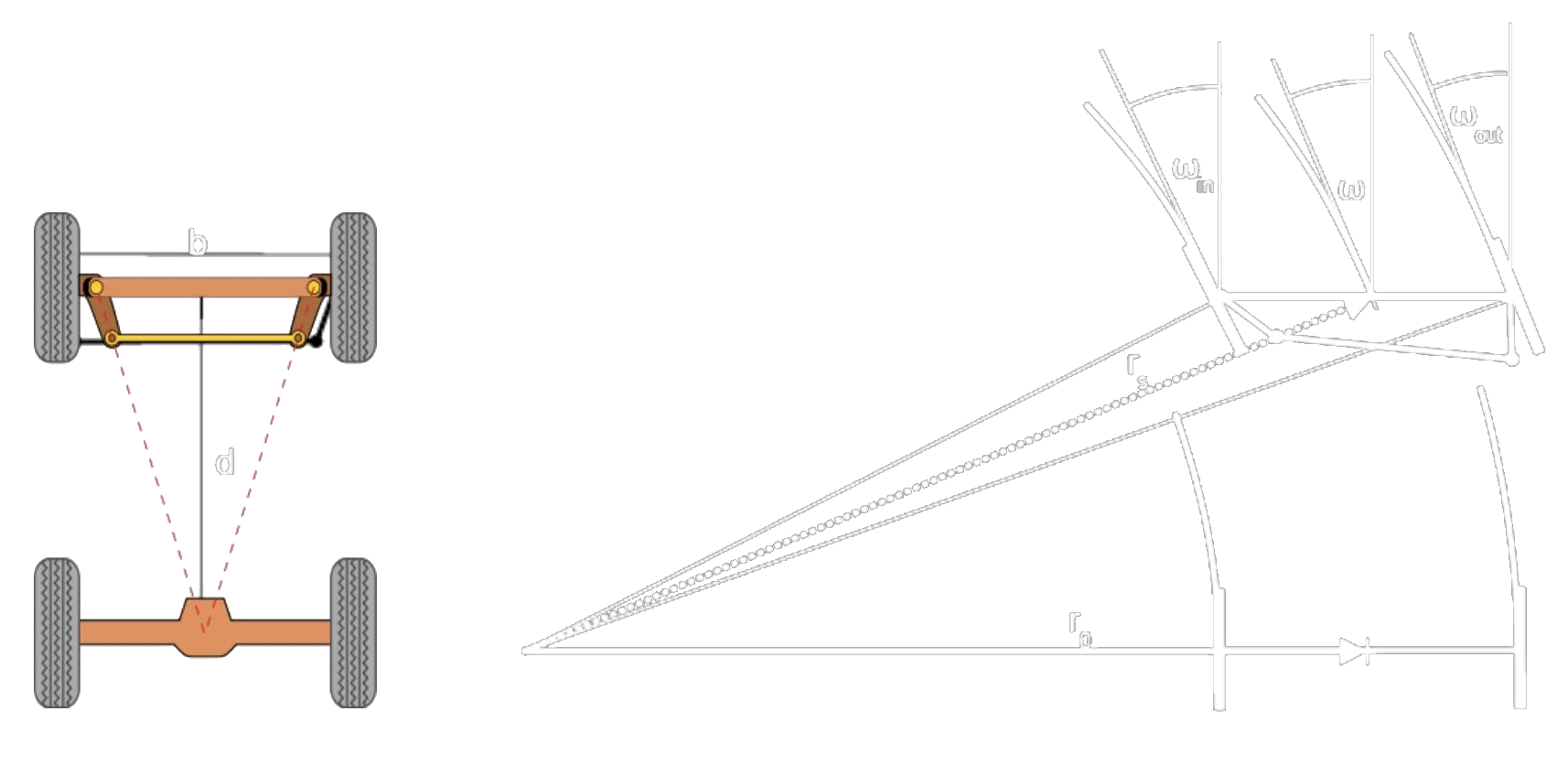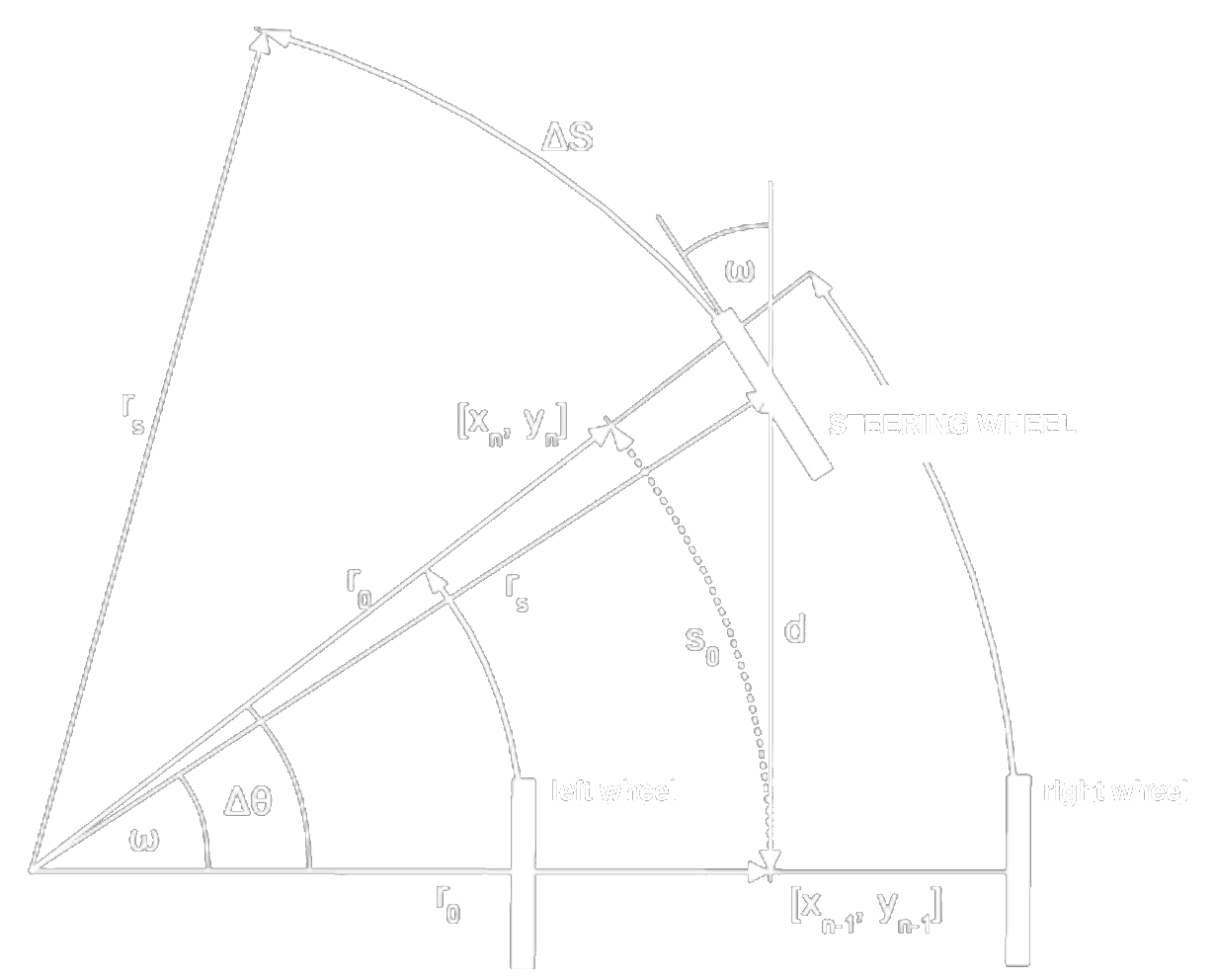Basic principle Simplified model
• Non-holonomic
• Constant $\omega \Rightarrow$ movement along a circle or straight line
• Circle: $r_{s}=\frac{d}{\sin \omega}, r_{0}=\frac{d}{\tg\omega}$
• Orientation change $\Delta \theta=\frac{\Delta S}{r_{s}}$
• Manoeuvring abilities depend on $d$ and $\omega_{\max }$ (wheelbase and wheel turn)

### Differential steering

• non-holonomic
$r_{n}=\frac{b}{2} \frac{\Delta R+\Delta L}{\Delta R-\Delta L}$
• Approximation:
$\Delta \theta =\frac{S_{n}}{r_{n}}=\frac{\frac{\Delta R+\Delta L}{2}}{\frac{b}{2} \frac{\Delta R+\Delta L}{\Delta R-\Delta L}}=\frac{\Delta R-\Delta L}{b}$ \begin{aligned} x_{n} & =x_{n-1}+S_{n}\left[\cos \left(\Delta \theta+\theta_{n-1}\right)\right] \\ y_{n} & =y_{n-1}+S_{n}\left[\sin \left(\Delta \theta+\theta_{n-1}\right)\right] \end{aligned}
• more precisely, integrating:
\begin{aligned} x(t)&=x_{0}+\frac{b\left(v_{r}+v_{l}\right)}{2\left(v_{r}-v_{l}\right)}\left[\sin \left(\left(v_{r}-v_{l}\right) t / b+\theta\right)-\sin (\theta)\right] \\ y(t)&=y_{0}+\frac{b\left(v_{r}+v_{l}\right)}{2\left(v_{r}-v_{l}\right)}\left[\cos \left(\left(v_{r}-v_{l}\right) t / b+\theta\right)-\cos (\theta)\right] \end{aligned}
• Differential steering for real life - General trajectory is replaced by a series of
• line segments $(\Delta R=\Delta L, r=\infty)$
• arcs $\left(\Delta R \neq \Delta L, r=\frac{b}{2} \frac{\Delta R+\Delta L}{\Delta R-\Delta L}\right)$
• Wisely set $\Delta t$
• Odometry

### Omnidirectional steering

• Wheels are able to run in any direction
• Swerve/Crab drive
• Omniwheel
• Mecanum wheel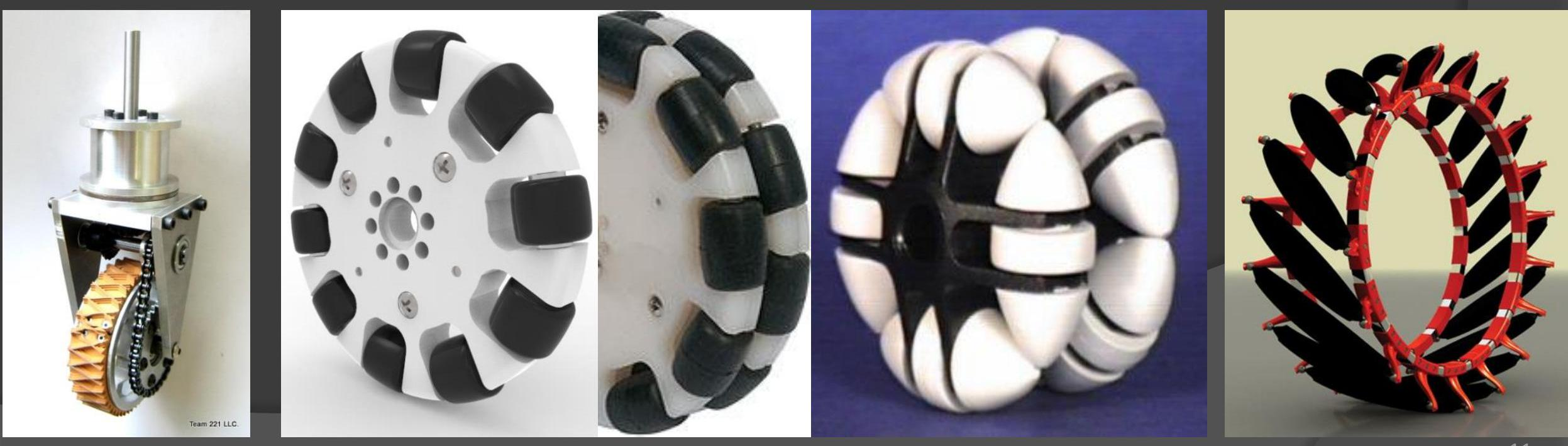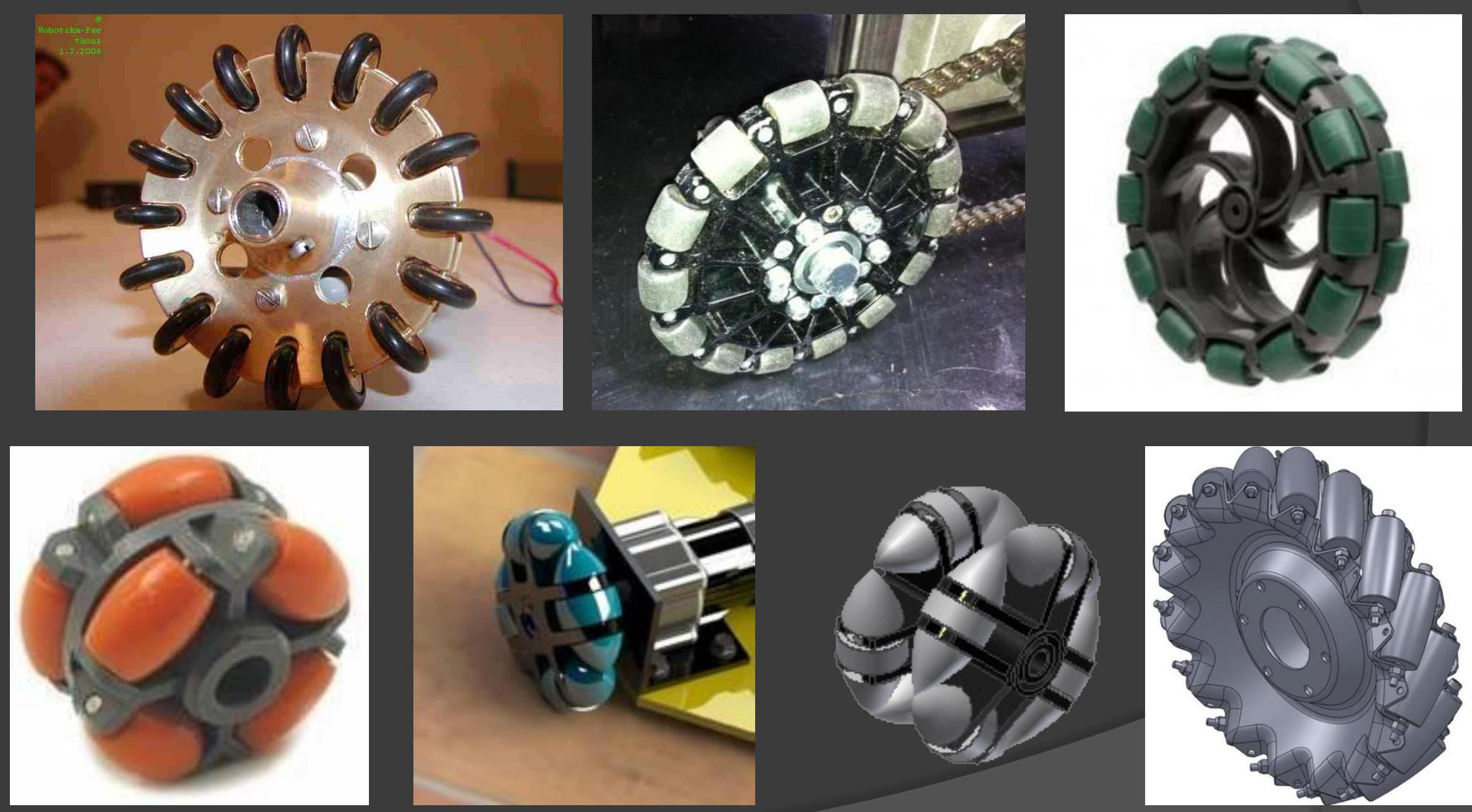• Syncro drive
• Holds yaw (body rotation) independently to movement direction
• Arbitrary movement as for omnidrive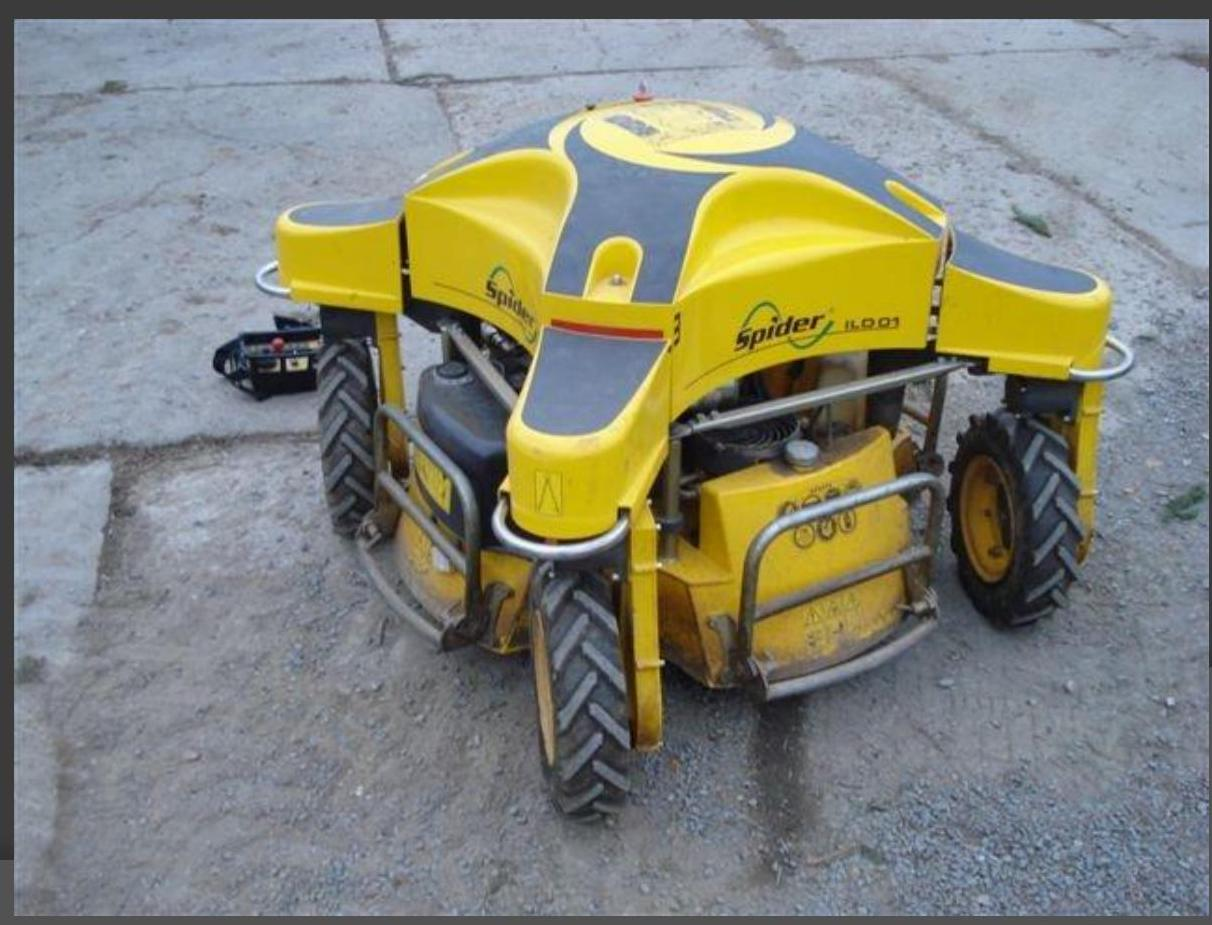• High manoeuvring ability
• sideways run
• start in any direction
• body orientation independent on movement direction
• on-place turning
• Simpler mechanical construction for omniwheels and mecanum wheels (fixed mount)

#### 1. Holonomic

• Movement easily calculated by vector combination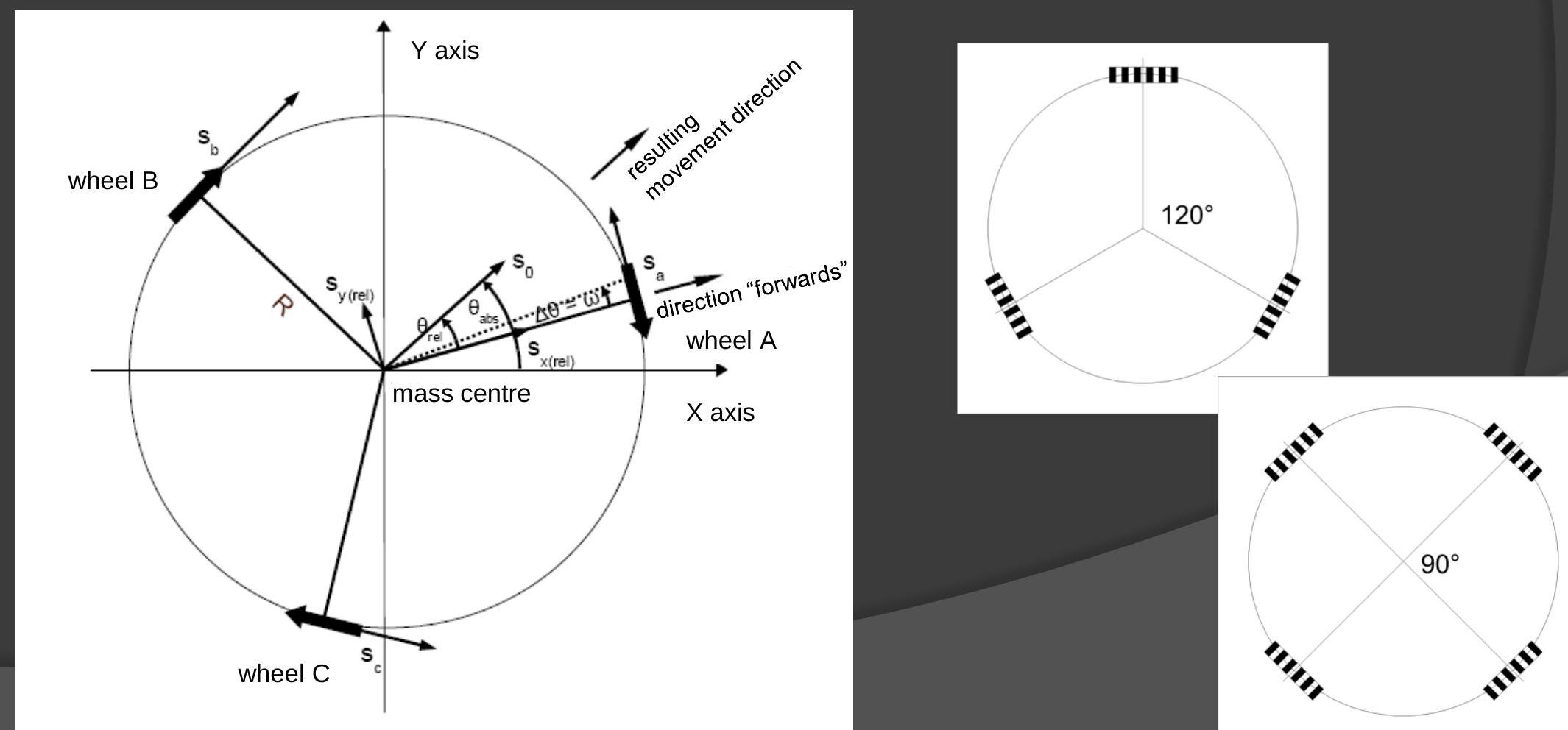#### 2. Killough / Ilon wheels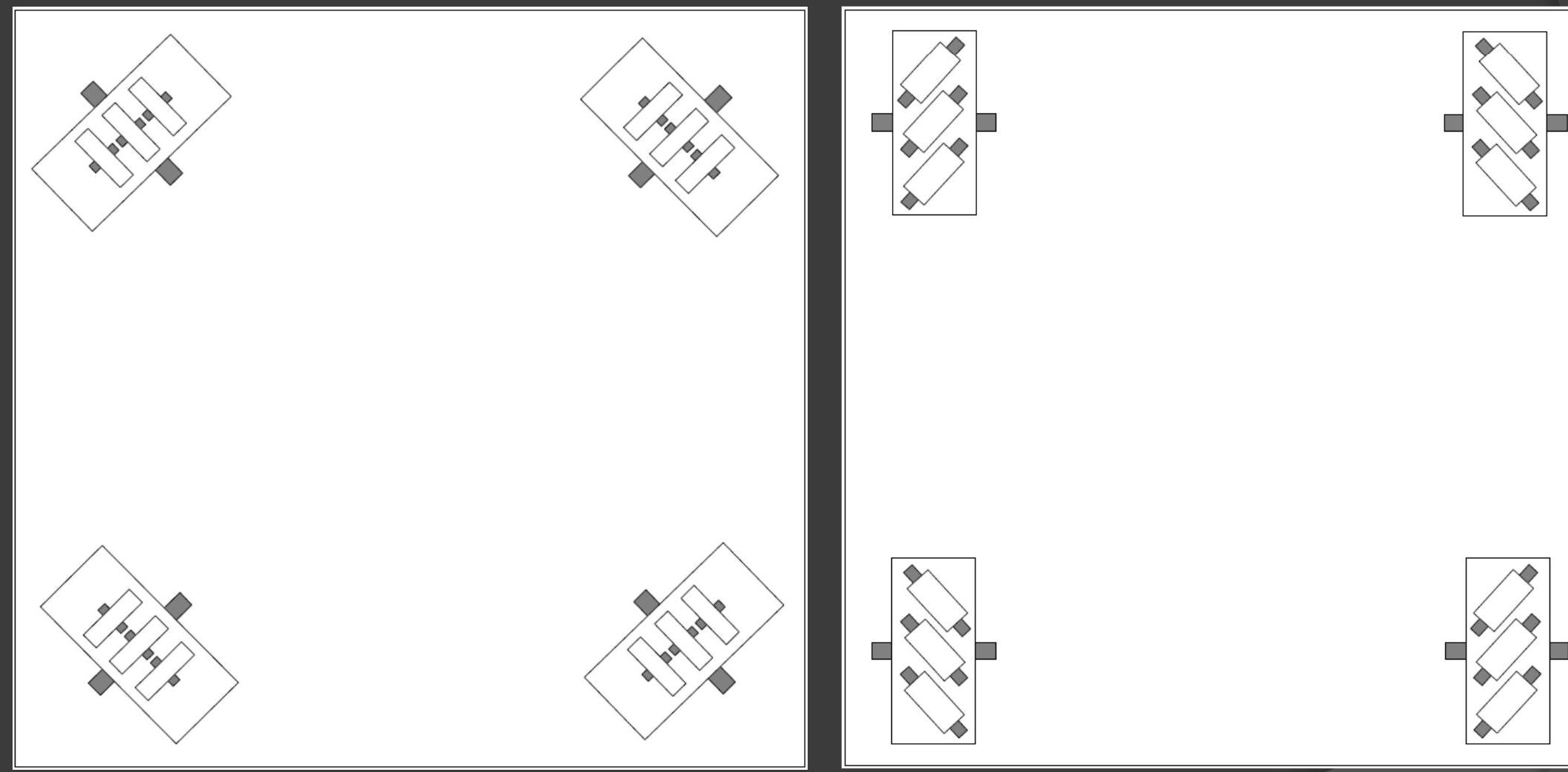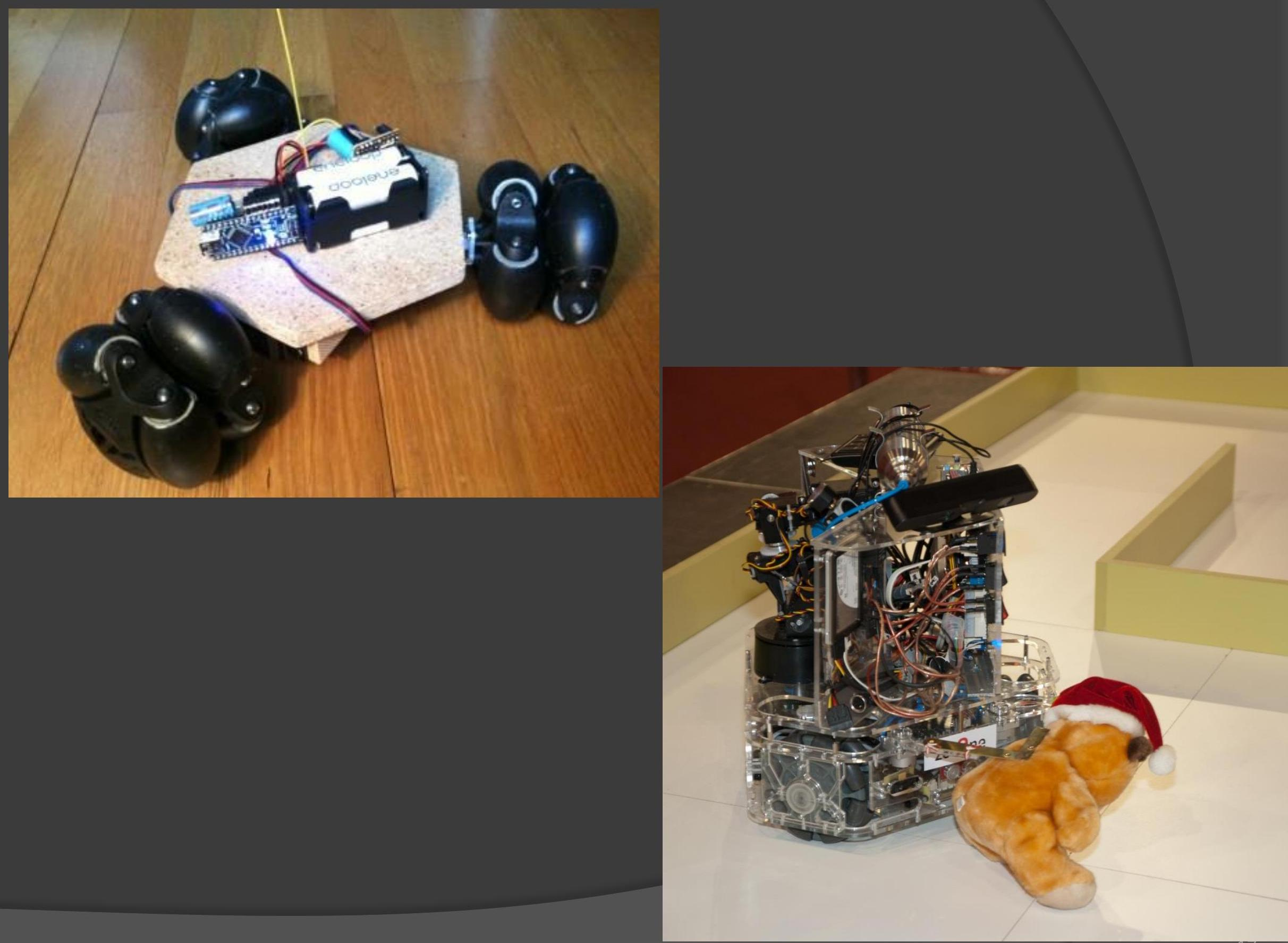#### 3. Omniwheel drive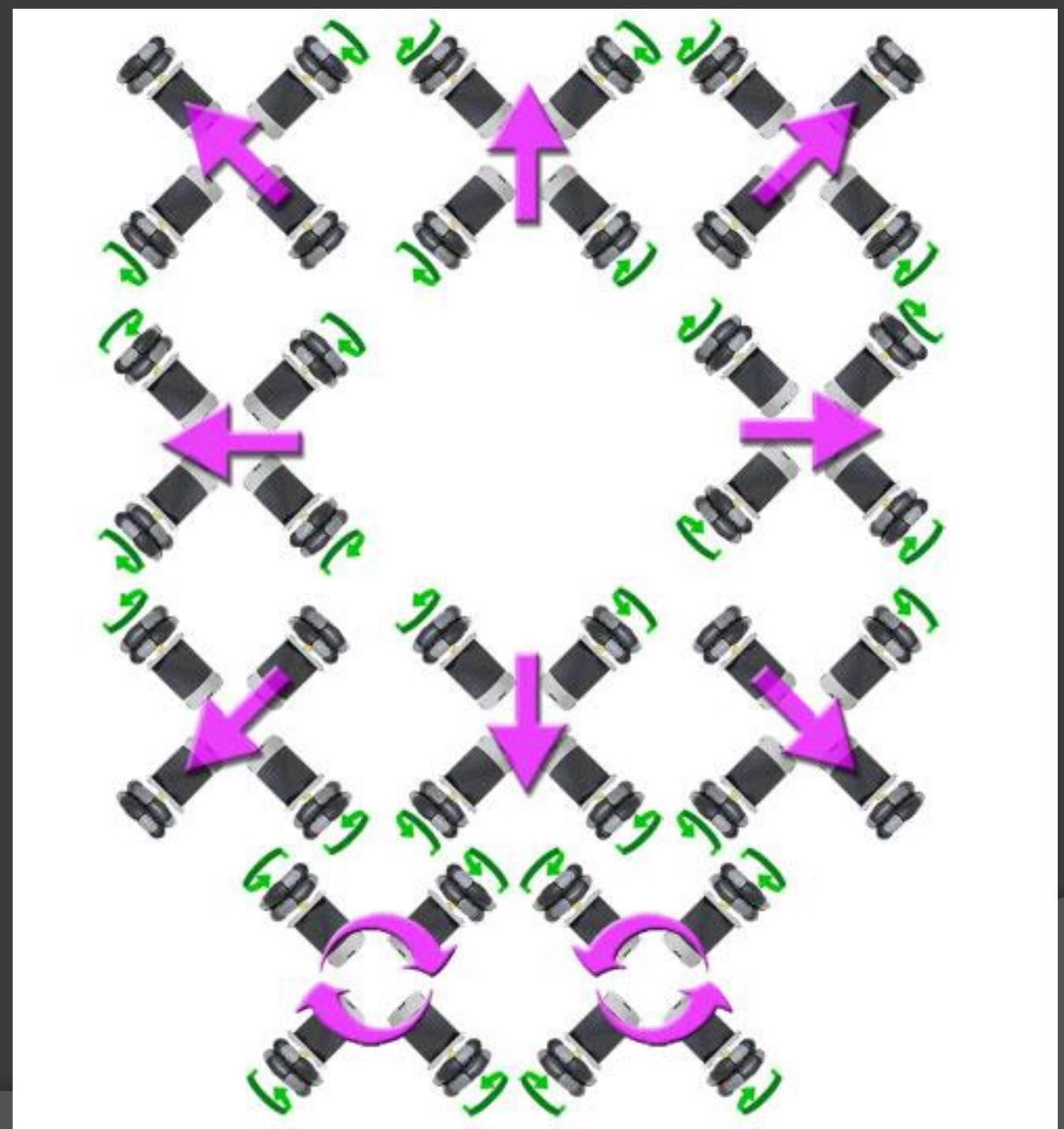#### Mecanum \& swerve drive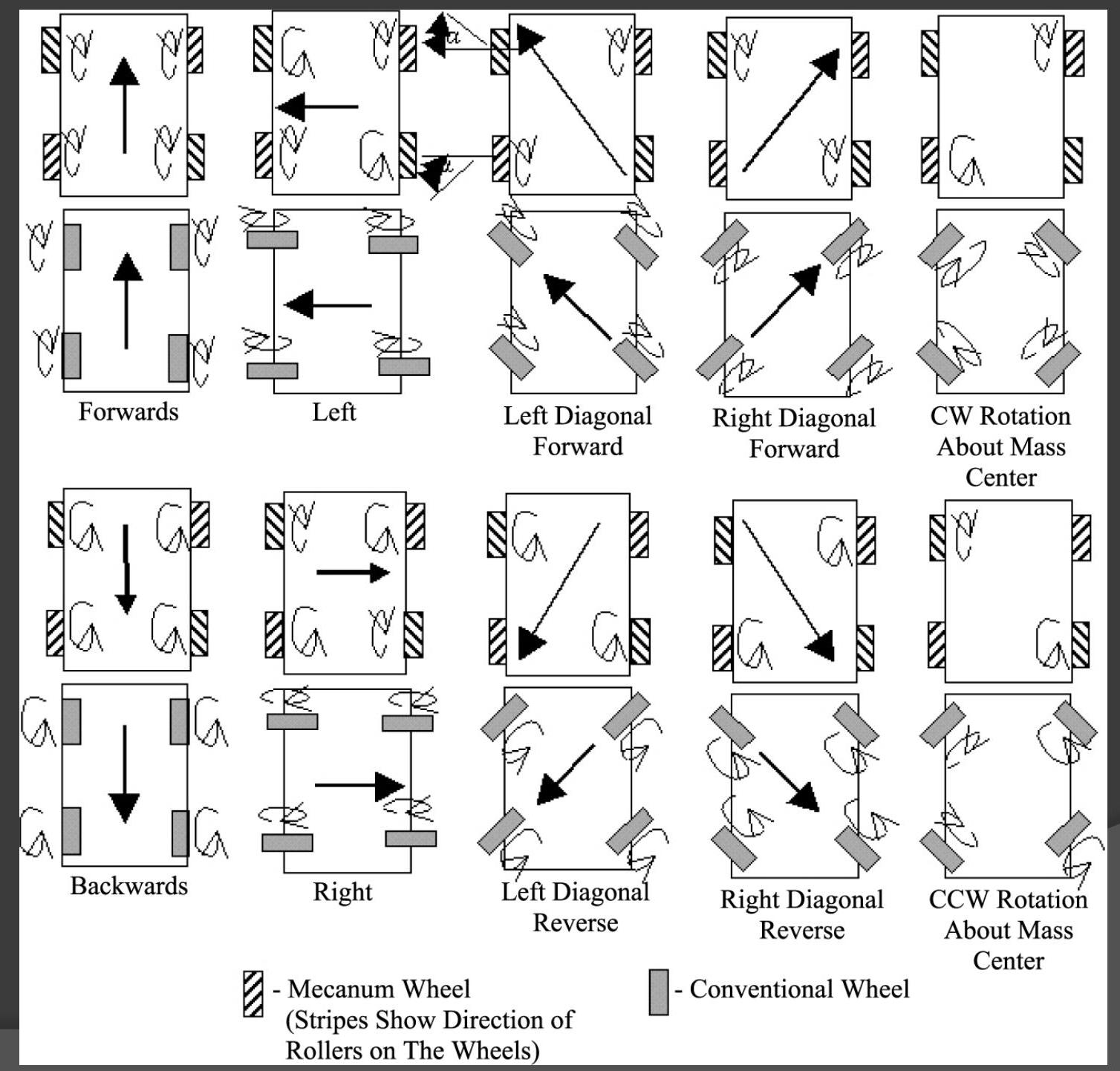### Robot movement

• Got $\overrightarrow{v_{t}}$ (translation speed) and $\vec{\omega}$ (rotation speeds)
• Need $\vec{v}$ - specific point speed
• vector approach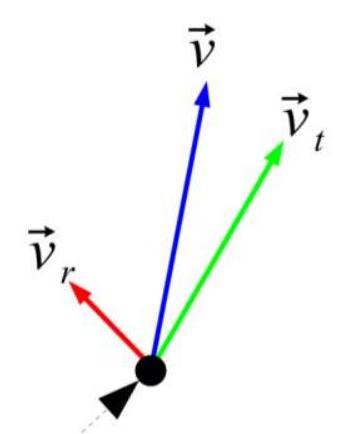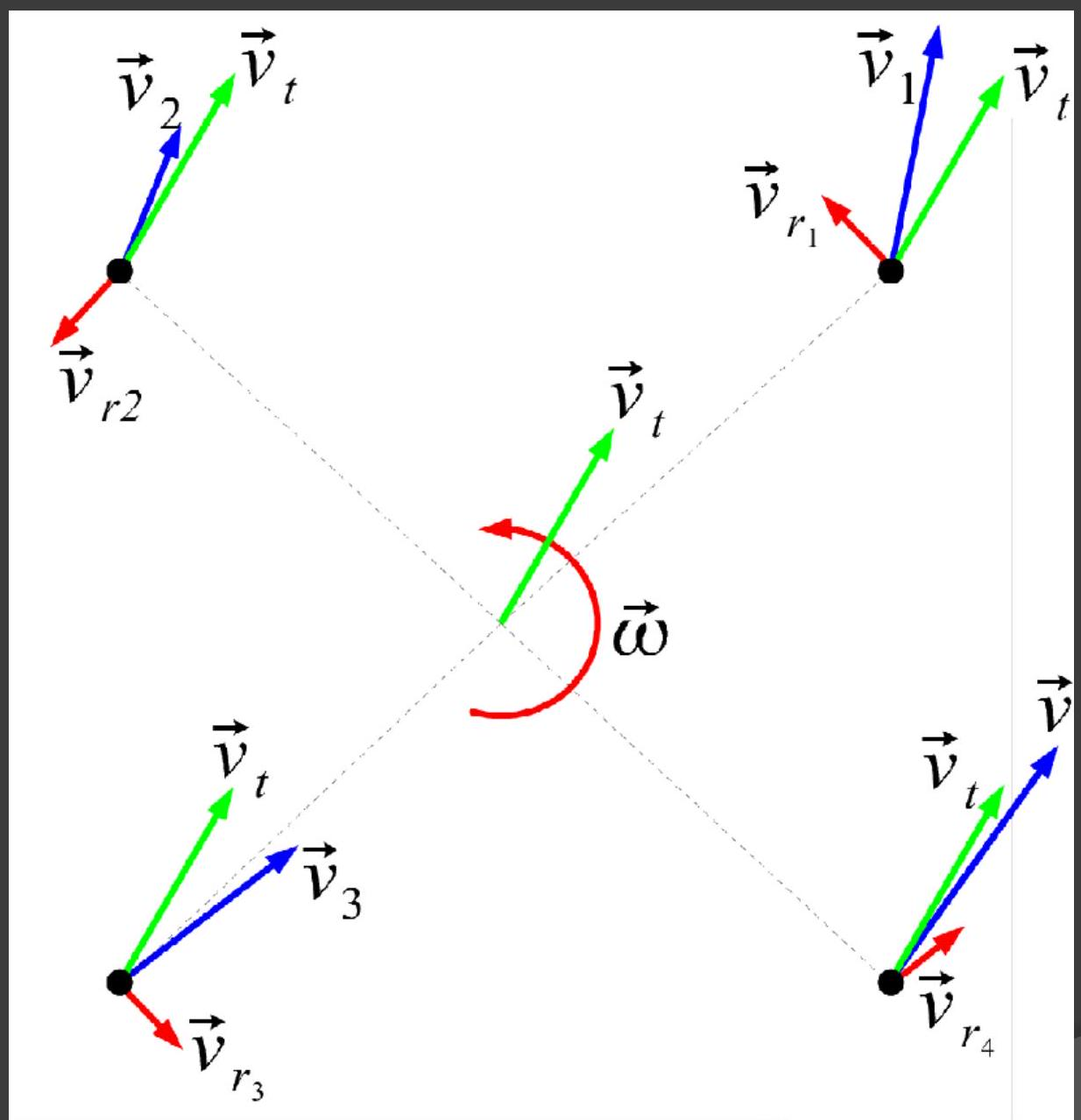#### Swerve drive

• Resolve $\overrightarrow{v_{t}}$ ($x$, $y$ components = axes velocities ) into wheel speed $v_{w}$ and steering angle $\theta$
\begin{aligned} v_{\omega} &=\sqrt{v_{x}^{2}+v_{y}^{2}} \\ \theta &=\arctan \left(\frac{v_{y}}{v_{x}}\right) \end{aligned}
• Resolve velocity into parallel and perpendicular components; magnitude $v$ of parallel component is wheel speed $v_{w}$
• $\hat{u}$ is a unit vector in the direction of the wheel (whichever direction is assumed to be “forwards”)
\begin{aligned} v_{w} &= v_{\|}=\vec{v} \cdot \hat{u} \\ &=\left(v_{x} \hat{\imath}+v_{y} \hat{\jmath}\right) \cdot\left(-\frac{1}{\sqrt{2}} \hat{\imath}+\frac{1}{\sqrt{2}} \hat{\jmath}\right) \\ &=-\frac{1}{\sqrt{2}} v_{x}+\frac{1}{\sqrt{2}} v_{y} \end{aligned}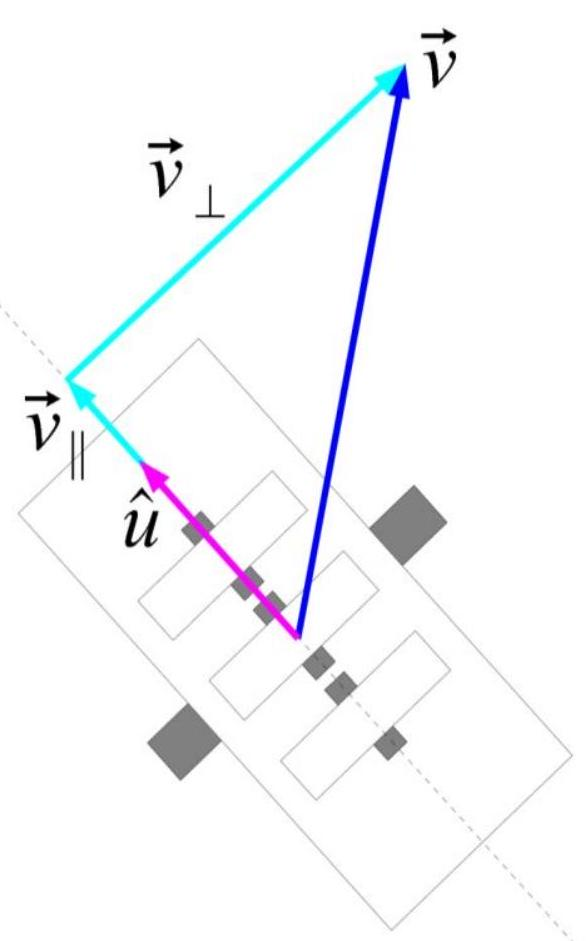#### Mecanum drive

• Similar to omniwheel drive
• Conceptually: Resolve velocity into components parallel to wheel and parallel to roller
• Not easy to calculate directly (directions are not perpendicular), so do it in two steps:
• Resolve to roller
• Resolve to wheel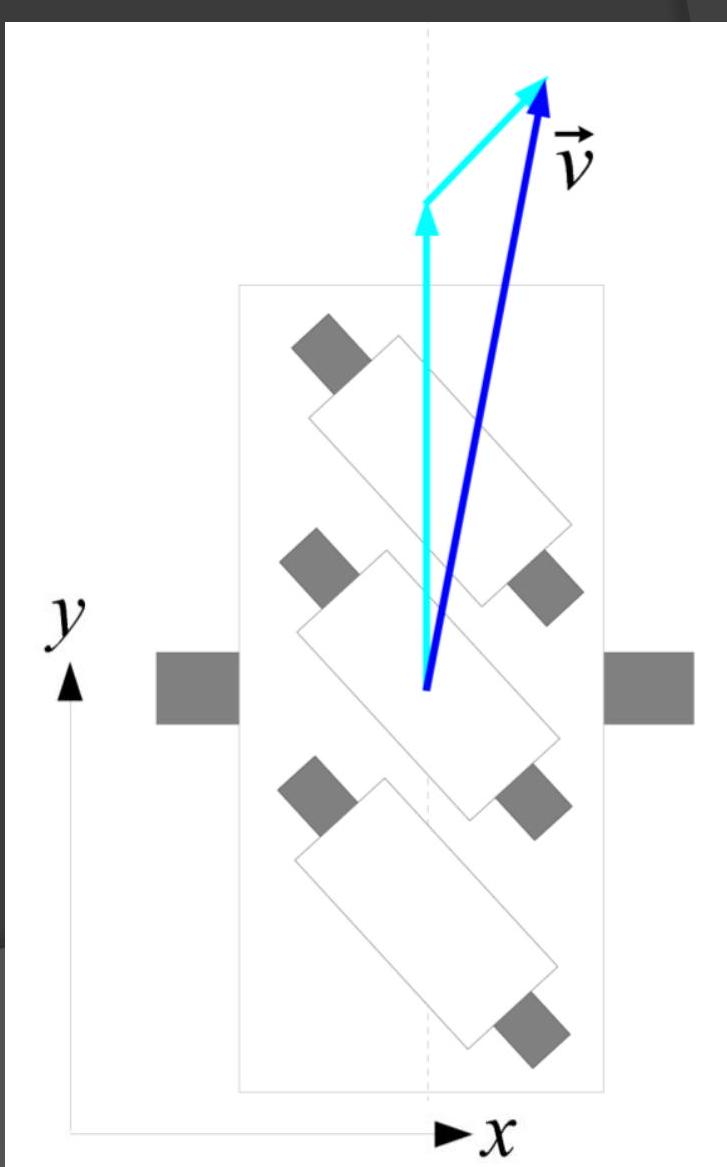• Resolve velocity into components parallel and perpendicular to roller axis
• $\hat{u}$ is not the same for each wheel; pick direction parallel to roller axis, in forwards direction
• Perpendicular component can be discarded
$v_{\|}=\vec{v} \cdot \hat{u}$ $=\left(v_{x} \hat{\imath}+v_{y} \hat{\jmath}\right) \cdot\left(-\frac{1}{\sqrt{2}} \hat{\imath}+\frac{1}{\sqrt{2}} \hat{\jmath}\right)$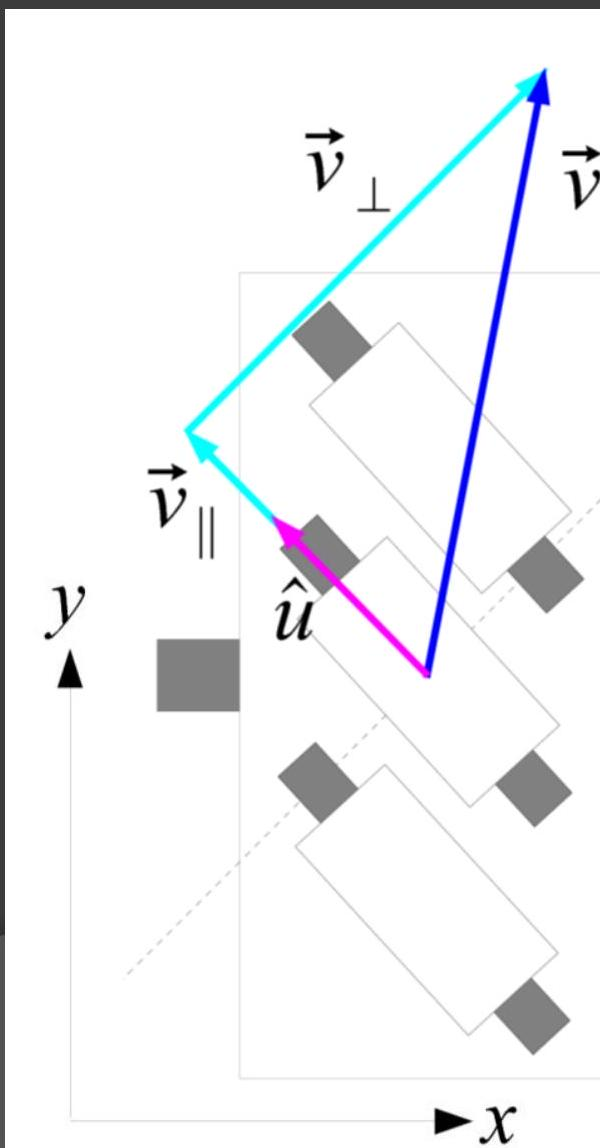• Use component parallel to roller axis and resolve it into components parallel to wheel and parallel to roller
• $v_{w}$ is the component parallel to the wheel
• When the angle is known, we can calculate $v_{w}$ directly.
• E.g. for $45^{\circ}$ inclination:
\begin{aligned} v_{w} & =\frac{v_{\|}}{\cos 45^{\circ}} \\ & =\sqrt{2}\left(-\frac{1}{\sqrt{2}} v_{x}+\frac{1}{\sqrt{2}} v_{y}\right) \\ & =-v_{x}+v_{y} \end{aligned}## Localization

• The goal is to find the position and orientation of the robot (pose)
1. relative to the map
2. relative to the environment
• Sometimes we also want to determine the position and orientation of individual parts

### Coordinate transformation

• The map is independent of the robot’s movement
• The sensors move with the robot - it has local coordinates
• Localization tries to map the local and global position onto each other
• The pose cannot be perceived directly
• We divide localization into
• absolute (global) / relative (local)
• passive / active
• static / dynamic (lighting, obstacles,… do (not) change )

### Absolute localization

• Measurement / Approximation regardless of previous state, typically more demanding on performance / technology
• Localization after reset / relocation is problematic (wake up / kidnap problem)
• Uses landmarks, or GPS and other services

### Relative localization

• Track position and update (odometry)
• we know the initial position
• we measure / estimate the change compared to the previous state (change in rotation and orientation)
• The problem is the accumulation of the error - after a long time the error can be too large

• the easiest localization
• direction + speed + time of movement
• accumulates an error

#### Odometry

• Measurement of wheel rotation (in different places we measure slightly different values) (TODO to explain)
• Systematic errors:
• losses
• other specified and actual parameters
• asymmetry
• measurement resolution
• sampling frequency
• etc.
• Unsystematic errors:
• terrain irregularities
• obstacles
• poor contact with the surface

#### Robot centric sensors

Most sensors move with the robot, so we have to do further data processing (e.g. recalculate coordinates relative to the global system,$\ldots$).

#### Types of localization

• Passive
• does not affect the control of the robot
• Active localization
• If the measurement is needed, it will affect the behavior of the robot
• Realistic localization
• Inputs are not trustworthy, actuators are not reliable, external influences must be taken into account

#### A possible solutions

1. More accurate measurement - better sensors and actuators, data filtering, the most accurate model of the robot and the environment
2. Do not rely on the fact that localization is accurate - probabilistic methods, fuzzy logic, interval algebra
• We can consider the data as random variables (inputs, pose)
• The position estimate is then a distribution
• Localization is then the search for a distribution that best matches the real position of the robot
• In 1D Belief $Bel(x)$ where $\int_{-\infty}^{\infty}Bel(x)dx=1$ Ideally, $Bel(x) = 1$ for exact but we can’t reach the position
• We want to model $Bel(x) = P(x\mid l_{1},\dots,l_{k})$
• Random modeling can be used with particle filters, Bayesian methods,…
• We estimate the posterior by measuring the prior
##### Representation
• Continuous representation is more accurate but harder to obtain and maintain (Kalman filter)
• That’s why we use a rather discrete representation
• This way we get probability grids, topological graphs,…
##### Probability Grid
• $Bel(l)$ indicates the probability of the appearance of a robot in the $L_{ij}$ field
• Difficulty depends on grid fineness and map size
• It can be reduced using
• selective updates (only interesting part of the grid)
• non-orthogonal graphs
##### Topological graphs
• Nodes - positions, edges - possible transitions
• $Bel(l)$ indicates the probability of occurrence in a given node
• More nodes can be made in the place where the robot is more likely to be found
• The chart does not need to contain geometric data
##### Monte Carlo localization
• A set of weighted points in space
• $Bel(l)$ is the sum of the weights of points at a given distance from l
• More points can be created for a more likely location
• Algorithm:
1. Sample movement, weights do not change
2. Correction, the positions of the points do not change, recalculate the weights
• Doesn’t work well for too accurate sensors, but not too imprecise either
• There is a lot of noise when navigating outside - MC can combine multiple measurements well
##### Continuous representation (Kalman filter)
• based on a normal distribution
• cannot record multiple hypotheses
• strict assumption: measurements must have a normal distribution
##### Other methods
• fuzzy logic
• interval algebra

#### Solution 3 no localization

• hardcoded automata
• reactive systems
• evolutionary algorithms
##### SLAM
• simultaneous location and mapping
• browsing a static environment
• we want to create a map and find the position from it
• a poorly recognized landmark can cause a huge error
• when using more landmarks, the difficulty increases ($n^2$, with $n \log n$ improvements)
• map errors and movement errors can be correlated
• knowledge of the angle with respect to two landmarks determines the part of the circle
• in addition, the distance can be calculated while moving

## Satellite localization

If we know the distance of the aiming point from the satellite, it will determine the sphere. Two specifies a circle and three a point. In practice, at least four satellites are typically used.

Simple idea: measuring the signal time-of-flight $\rightarrow$ distance

### History

• Werner von Braun (V1, V2,…)
• Sputnik(1957) The Doppler effect on the signal was another idea for a method of measurement.
• Transit
• US Navy
• proof of concept, but didn’t cover the whole country, slow, 2D only
• TODO technology
• Timeation
• uses precise (later atomic) clocks
• 2D only
• 621B
• US air force
• Can also target planes (3D)
• Pseudo-random noise increases resilience
• Needs constant satellite-ground connection
• NAVSEG
• a group formed by the cooperation of several sectors
• Uses ideas from Transit (orbit + prediction) and Timetion (accurate clock) and 621B (resilient signal)
• In 1973, Navstar GPS was created, the basis of today’s GPS launched in 1978
• Initially intended only for the military, later a less accurate version of the signal was broadcast for civilian purposes.
• Since 2000, even the more accurate army version is available to everyone
• Navigation failure caused several air accidents (typically wrong trajectory -> shot down USSR)

### GPS satellites

• 6 Orbit $60{\degree}$ gap declination $55\degree$ after four slots
• There are at least four satellites in each orbit
• At least 4 satellites are visible from every location on earth
• Due to atmospheric phenomena and calculation errors, the GPS error is about 15m with post processing, better accuracy around 10m can be achieved

### Competitive global systems

Compass(China), Galileo(EU), GLONASS(Russia)

### Regional systems

Beidou(China), DORIS(France), IRNSS(India)

### Local systems

• EGNOS, GAGAN, MSAS, WAAS
• Typically a stationary satellite

### Galileo

European project, more accurate (about 1 m)

### Ublox

• centimeter accuracy
• uses RTK technology, which combines several techniques and thus achieves higher accuracy (verify)

### Indoor GPS

• Local location determination can be done using Wifi or bluetooth
• More accurate technology can achieve about 1m accuracy

## Environment representation

### Maps

we divide according to several aspects

• according to production
• manual (expensive)
• (semi)automatic
• According to production time
• online
• offline
• According to time of use
• Firm
• basic division
• Metric
• Topological
• By level of abstraction
• Sensory
• Geometric
• Topological
• Symbolic

### Types of maps

• Metric maps
• Based on the given coordinate system
• Objects are represented by coordinates
• tourist map, (city plan)…
• Topological maps
• They don’t have positional information only about relationships between objects.
• For example connections, neighboring objects…
• Additional information: names, attributes and other data important to the robot.
• Typically chart + labels + metadata.
• Maps in robotics tend to be a combination of both approaches.
• Sensory maps
• Uses sensor input or pre-processed data (filtered,…).
• For example occupancy grid - regular (square, hex,…) or irregular (various sizes and shapes).
• Mostly they are only local
• Geometric maps
• Description based on geom objects: curves, cubes, cylinders,…
• Automatic production is demanding
• Topological maps
• typically a geom map generalized to a graph
• mostly used by:
• nodes = objects and edges = adjacent obj
• nodes = objects and connections, edges = affiliations (TODO meaning)
• Symbolic maps
• for direct robot communication with people, e.g. prolog(?)
• Occupancy grid
• directly it is difficult to maintain
• probabilistic methods
• fuzzy approach
• Neurons, genetic alg. - a problem with learning and anticipating unexpected events

## Planning

### Prerequisites

• Mapping
• Knowledge of location and destination
• Movement model
• Metric

#### Description of the problem

• go from start to finish
• find a path or notice that it does not exist
• reacts to the surroundings - avoiding obstacles,…
• Sometimes we want navigation: planning and avoiding obstacles
• Planning is the opposite of avoiding obstacles, however both complement each other

### Scheduling algorithms

• Graph methods
• Use of grid, potential field
• independent of map type
• Exact, Approximate, Probabilistic, $\ldots$

### Bug algorithm

• has no global model - no map, does not know the location of obstacles or if there is a solution
• knows the destination location relative to the starting location
• only local detection of the surroundings (contact), possibly at a short distance
• Reactive

#### Prerequisites bug alg

• 2D static environment
• Final parameters: the number of obstacles and their perimeters,
• non-zero thickness of obstacles
• Straight line crosses with obstacles
• Obstacles do not touch, they can be united when overlapping

#### Bug 0

• Go straight to the target
• When you hit an obstacle, go around the obstacle until you can’t go straight to the goal
• It may not work - for example, for a circle with a small hole (where the target is on the opposite side to the center), the robot will go inside and before it comes out, it will get to the state where it can go to the target again

#### Bug 1

• Go straight to the target
• When you hit an obstacle, go around the obstacle
• After you go around the obstacle, go to the place that was closest to the goal and go straight again
• The robot will never return to the obstacle from which it left (it will exit from the place that is closest to the target towards it)
• Because the robot’s position is always in a sufficiently large circle around the target, it will encounter only finitely many obstacles, so the algorithm is finite.
• The robot does not ignore obstacles, so the paths found are valid
• If the path existed but the robot did not find it, then the path from the minimum to the goal must lead directly to the obstacle
• But each straight line passes through the obstacle $2k$ times. Therefore there is another intersection, this intersection must be closer to the goal because it lies on the way to the goal. If there is a path to this place, the robot would choose this - the dispute cannot get closer to the goal. (TODO check)

• Distance Estimate
• Best case $\lvert \text{target} - \text{start} \rvert$
• Worst case
• For each obstacle in the circle $\lvert \text{target}-\text{start}\rvert$ from the target, we count 1.5 times the perimeter (he goes around it and chooses a shorter path to the minimum) + $\lvert \text{target}-\text{start}\rvert$

#### Bug 2

Go to the goal, if you come across an obstacle, go around it until you can’t go straight to the goal again

Lemma

Bug 2 finally encounters many obstacles and passes them all through the start-finish junction

Bug 2 passes each point on the obstacle at most $\frac{n_i}{2}$ times, where $n_i$ is the number of crossings of the obstacle $i$ with the start-finish line.

• Distance Estimate
• Best case $\lvert \text{target} - \text{start} \rvert$
• Worst case - For each obstacle on the connecting line between the start and the finish line, we count half the circumference
$\lvert \text{target} - \text{start} \rvert + \sum_{i=1}^{N}\frac{n_i\cdot p_i}{2}$

#### Comparison of Bug 1 and Bug 2 (TODO)

There are cases for which 1 is better than two and vice versa

Estimates for length can be improved if the robot can see some of its surroundings (area inside the circle around itself)

#### Tangent bug

selects by angle of direction to direction to target (TODO) It is correct

### Dijkstra

alternative path search A* is a Dijkstra + heuristic, if the heuristic satisfies something it can be proven that it does not process vertices twice. E.g. price + distance from destination D* also handles replanning when an obstacle appears, starting from the destination

### Rapidly exploring random trees

Randomized algorithm It quickly finds some solution and iteratively adjusts

### Potential field

The robot is in sweat. fields, obstacles are peaks - repel the goal is the minimum

## Multirobot systems

### Why use multirobot systems

• Too challenging for one robot
• The task is naturally distributed
• One universal robot would be too complex, more specialized ones are easier to make
• Parallelism accelerates the solution of the task
• Greater robustness
• Application
• Warehouses
• Modeling the real world
• A swarm of robots
• robots can cooperate or act independently

### Types of systems

• Centralized Architecture
• One control center
• Possible but problematic: the failure of the center may mean the failure of all robots, too much communication load on the center.
• Hierarchical Architecture
• Divide and rule
• Robots have small groups of robots that he gives orders to
• Scales better, problematic when higher level control fails
• Decentralized Architecture
• robots act according to (semi) local perceptions
• Every robot must know high level target, problematic target changes
• Hybrid driving
• combination of local control with control of multiple robots (TODO check)

## Satellite localization

If we know the distance of the aiming point from the satellite, it will determine the sphere. Two specifies a circle and three a point. In practice, at least four satellites are typically used.

Simple idea: measuring the signal time-of-flight $\rightarrow$ distance

### History

• Werner von Braun (V1, V2,…)
• Sputnik(1957) The Doppler effect on the signal was another idea for a method of measurement.
• Transit
• US Navy
• proof of concept, but didn’t cover the whole country, slow, 2D only
• TODO technology
• Timeation
• uses precise (later atomic) clocks
• 2D only
• 621B
• US air force
• Can also target planes (3D)
• Pseudo-random noise increases resilience
• Needs constant satellite-ground connection
• NAVSEG
• a group formed by the cooperation of several sectors
• Uses ideas from Transit (orbit + prediction) and Timetion (accurate clock) and 621B (resilient signal)
• In 1973, Navstar GPS was created, the basis of today’s GPS launched in 1978
• Initially intended only for the military, later a less accurate version of the signal was broadcast for civilian purposes.
• Since 2000, even the more accurate army version is available to everyone
• Navigation failure caused several air accidents (typically wrong trajectory $\rightarrow$ shot down USSR)

### GPS satellites

• 6 Orbit $60{\degree}$ gap declination $55\degree$ after four slots
• There are at least four satellites in each orbit
• At least 4 satellites are visible from every location on earth
• Due to atmospheric phenomena and calculation errors, the GPS error is about 15m with post processing, better accuracy around 10m can be achieved

### Competitive global systems

Compass(China), Galileo(EU), GLONASS(Russia)

### Regional systems

Beidou(China), DORIS(France), IRNSS(India)

### Local systems

• EGNOS, GAGAN, MSAS, WAAS
• Typically a stationary satellite

### Galileo

European project, more accurate (about 1 m)

### Ublox

• centimeter accuracy
• uses RTK technology, which combines several techniques and thus achieves higher accuracy (verify)

### Indoor GPS

• Local location determination can be done using Wifi or bluetooth
• More accurate technology can achieve about 1m accuracy